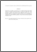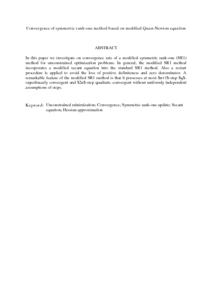# Convergence of symmetric rank-one method based on modified Quasi-Newton equation

## Citation

Khiyabani, Farzin Modarres and Abu Hassan, Malik and Leong, Wah June (2010) Convergence of symmetric rank-one method based on modified Quasi-Newton equation. Journal of Mathematics Research, 2 (3). pp. 97-102. ISSN 1916-9795; ESSN: 1916-9809

## Abstract

In this paper we investigate on convergence rate of a modified symmetric rank-one (SR1) method for unconstrained optimization problems. In general, the modified SR1 method incorporates a modified secant equation into the standard SR1 method. Also a restart procedure is applied to avoid the loss of positive definiteness and zero denominator. A remarkable feature of the modified SR1 method is that it possesses at most \$n+1\$-step \$q\$-superlinearly convergent and \$2n\$-step quadratic convergent without uniformly independent assumptions of steps.Preview
PDF (Abstract)
Convergence of symmetric rank.pdfView Item